Anzeige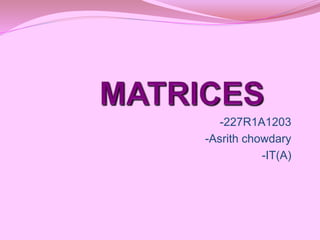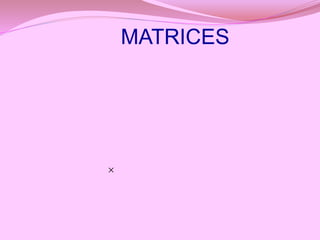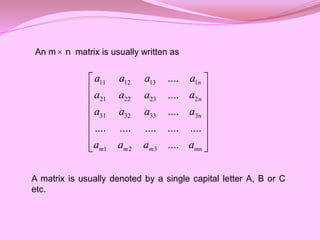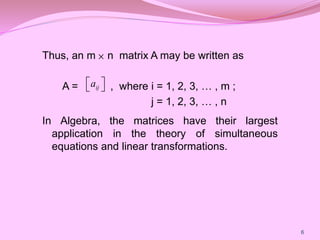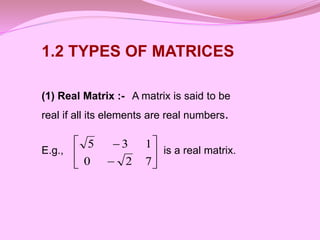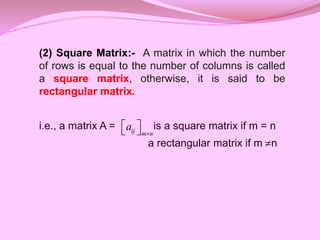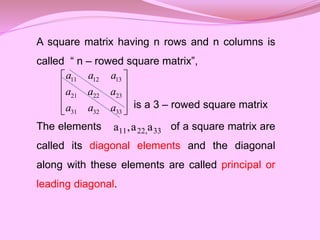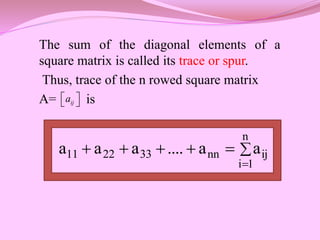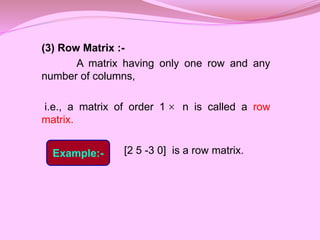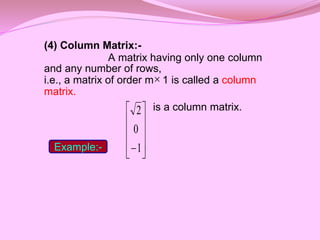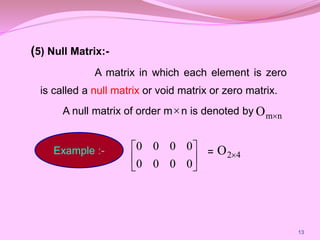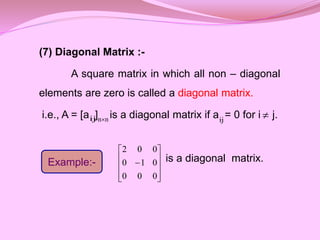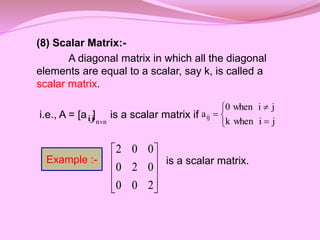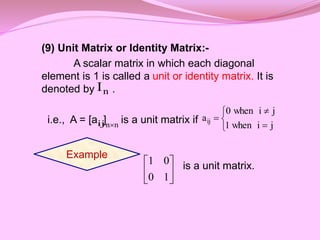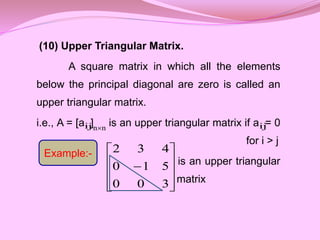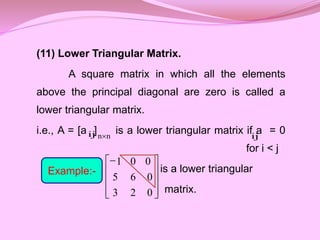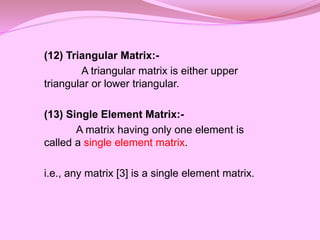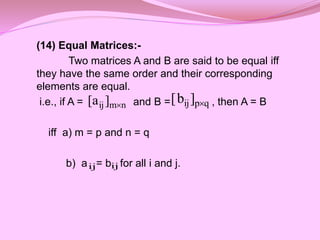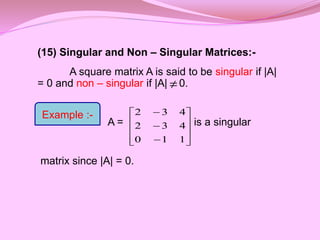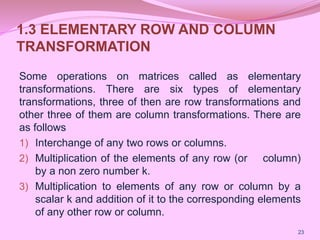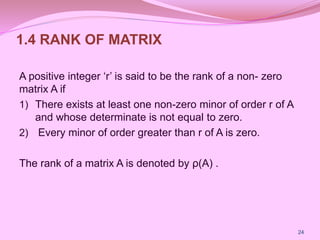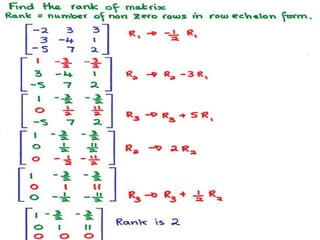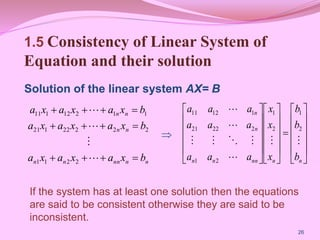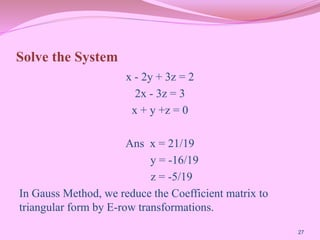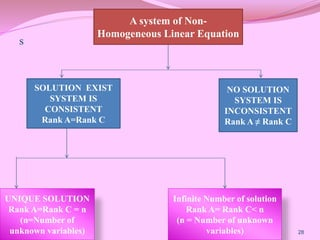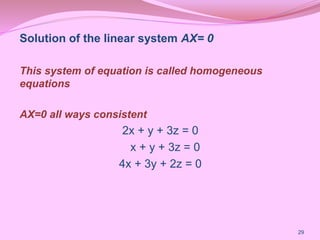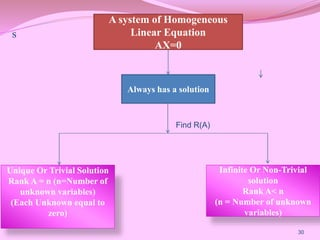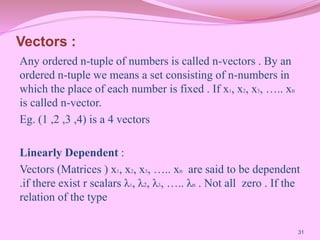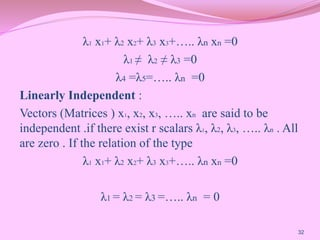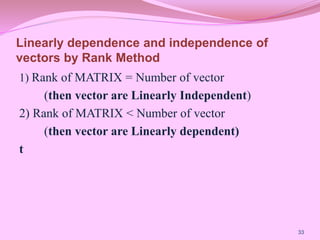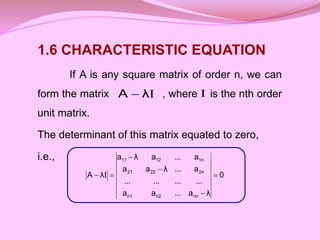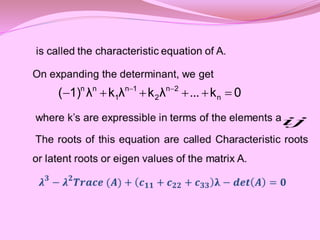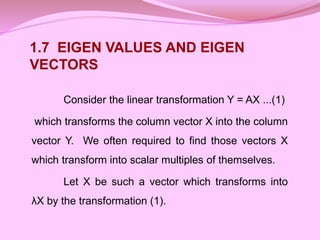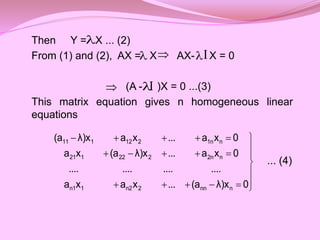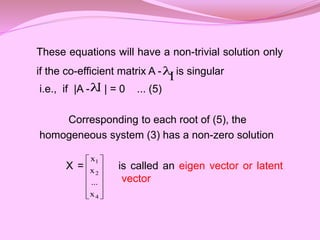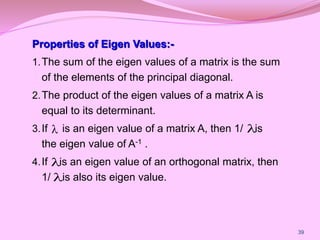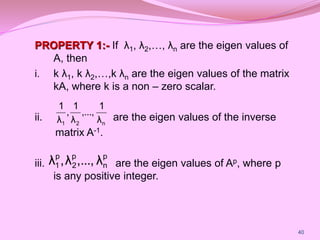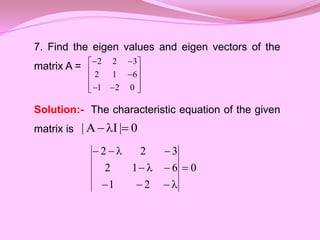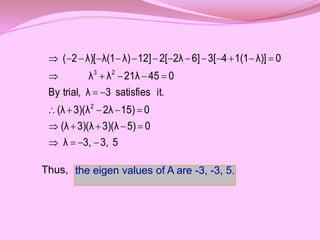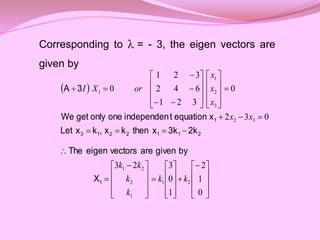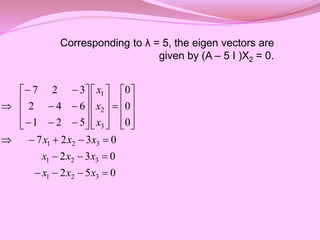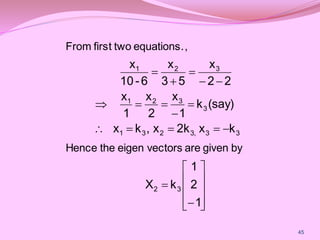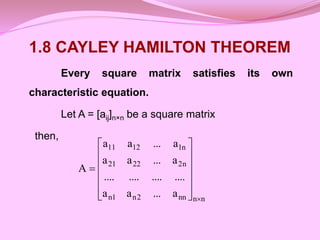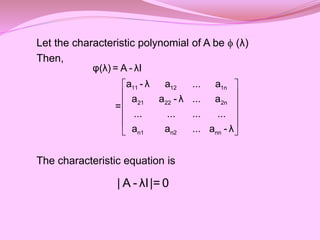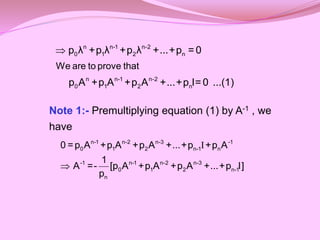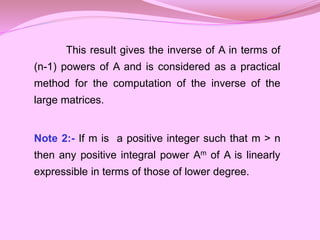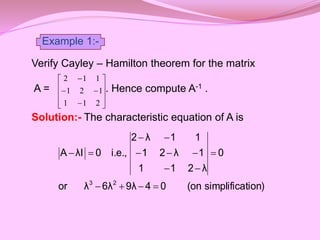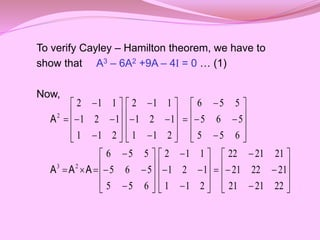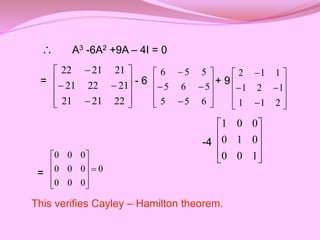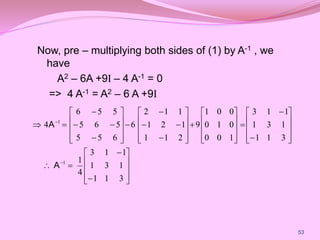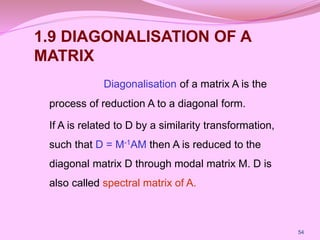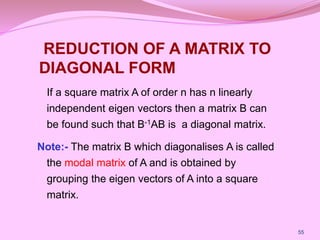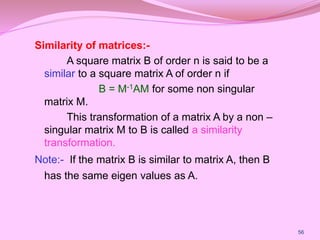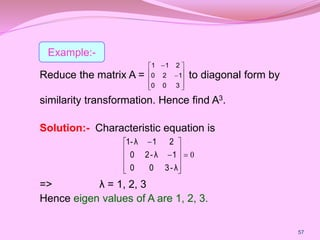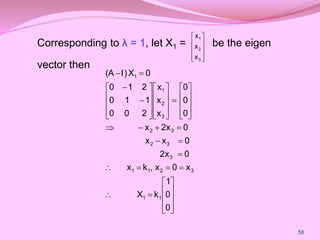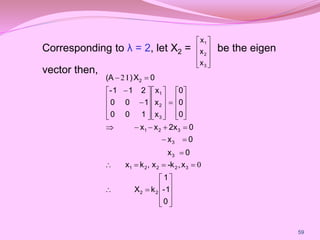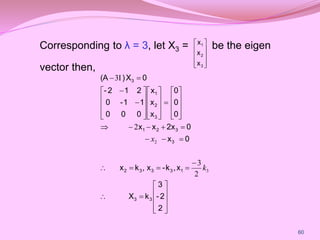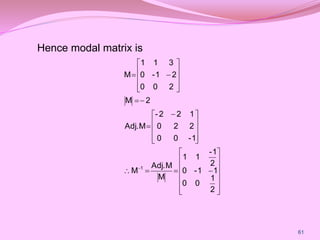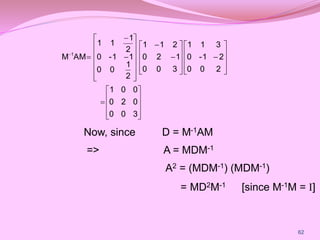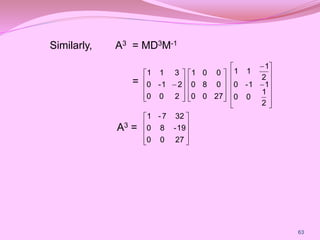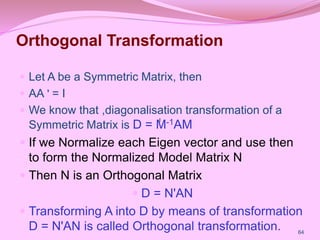1 von 65
Anzeige

### Matrix_PPT.pptx

1. -227R1A1203 -Asrith chowdary -IT(A)
2. MATRICES CONTENT 1.1 INTRODUCTION 1.2 TYPES OF MATRICES 1.3 ELEMENTARY ROW AND COLUMN TRANSFORMATION 1.4 RANK OF MATRIX 2
3. 3
4.  MATRICES
5. An m n matrix is usually written as A matrix is usually denoted by a single capital letter A, B or C etc. 11 12 13 1 21 22 23 2 31 32 33 3 1 2 3 .... .... .... .... .... .... .... .... .... n n n m m m mn a a a a a a a a a a a a a a a a                 
6. Thus, an m n matrix A may be written as A = , where i = 1, 2, 3, … , m ; j = 1, 2, 3, … , n In Algebra, the matrices have their largest application in the theory of simultaneous equations and linear transformations.  6 ij a    
7.         7 2 0 1 3 5 1.2 TYPES OF MATRICES (1) Real Matrix :- A matrix is said to be real if all its elements are real numbers. E.g., is a real matrix.
8. (2) Square Matrix:- A matrix in which the number of rows is equal to the number of columns is called a square matrix, otherwise, it is said to be rectangular matrix. i.e., a matrix A = is a square matrix if m = n a rectangular matrix if m n ij m n a      
9. A square matrix having n rows and n columns is called “ n – rowed square matrix”, is a 3 – rowed square matrix The elements of a square matrix are called its diagonal elements and the diagonal along with these elements are called principal or leading diagonal. 11 12 13 21 22 23 31 32 33 a a a a a a a a a           33 , 22 11 a a , a
10. The sum of the diagonal elements of a square matrix is called its trace or spur. Thus, trace of the n rowed square matrix A= is ij a            n 1 i ij nn 33 22 11 a a .... a a a
11. (3) Row Matrix :- A matrix having only one row and any number of columns, i.e., a matrix of order 1 n is called a row matrix. [2 5 -3 0] is a row matrix.  Example:-
12. (4) Column Matrix:- A matrix having only one column and any number of rows, i.e., a matrix of order m 1 is called a column matrix. is a column matrix.           1 0 2  Example:-
13. (5) Null Matrix:- A matrix in which each element is zero is called a null matrix or void matrix or zero matrix. A null matrix of order m n is denoted by = 13  n m O  Example :-       0 0 0 0 0 0 0 0 4 2 O 
14. (6) Sub - matrix :- A matrix obtained from a given matrix A by deleting some of its rows or columns or both is called a sub – matrix of A. is a sub – matrix of       4 1 0 3             2 4 6 1 7 0 5 3 5 2 1 0 Example:-
15. (7) Diagonal Matrix :- A square matrix in which all non – diagonal elements are zero is called a diagonal matrix. i.e., A = [a ] is a diagonal matrix if a = 0 for i j. is a diagonal matrix. ij n n ij  Example:-            0 0 0 0 1 0 0 0 2
16. (8) Scalar Matrix:- A diagonal matrix in which all the diagonal elements are equal to a scalar, say k, is called a scalar matrix. i.e., A = [a ] is a scalar matrix if is a scalar matrix.           2 0 0 0 2 0 0 0 2 ij n n       j i when k j i when 0 aij Example :-
17. (9) Unit Matrix or Identity Matrix:- A scalar matrix in which each diagonal element is 1 is called a unit or identity matrix. It is denoted by . i.e., A = [a ] is a unit matrix if is a unit matrix. n I ij n n       j i when 1 j i when 0 aij Example       1 0 0 1
18. (10) Upper Triangular Matrix. A square matrix in which all the elements below the principal diagonal are zero is called an upper triangular matrix. i.e., A = [a ] is an upper triangular matrix if a = 0 for i > j is an upper triangular matrix            3 0 0 5 1 0 4 3 2 ij n n ij Example:-
19. (11) Lower Triangular Matrix. A square matrix in which all the elements above the principal diagonal are zero is called a lower triangular matrix. i.e., A = [a ] is a lower triangular matrix if a = 0 for i < j is a lower triangular matrix. ij n n ij Example:-           0 2 3 0 6 5 0 0 1
20. (12) Triangular Matrix:- A triangular matrix is either upper triangular or lower triangular. (13) Single Element Matrix:- A matrix having only one element is called a single element matrix. i.e., any matrix  is a single element matrix.
21. (14) Equal Matrices:- Two matrices A and B are said to be equal iff they have the same order and their corresponding elements are equal. i.e., if A = and B = , then A = B iff a) m = p and n = q b) a = b for all i and j. q p ij] b [  n m ij] a [  ij ij
22. (15) Singular and Non – Singular Matrices:- A square matrix A is said to be singular if |A| = 0 and non – singular if |A| 0. A = is a singular matrix since |A| = 0.              1 1 0 4 3 2 4 3 2  Example :-
23. 1.3 ELEMENTARY ROW AND COLUMN TRANSFORMATION Some operations on matrices called as elementary transformations. There are six types of elementary transformations, three of then are row transformations and other three of them are column transformations. There are as follows 1) Interchange of any two rows or columns. 2) Multiplication of the elements of any row (or column) by a non zero number k. 3) Multiplication to elements of any row or column by a scalar k and addition of it to the corresponding elements of any other row or column. 23
24. 1.4 RANK OF MATRIX A positive integer ‘r’ is said to be the rank of a non- zero matrix A if 1) There exists at least one non-zero minor of order r of A and whose determinate is not equal to zero. 2) Every minor of order greater than r of A is zero. The rank of a matrix A is denoted by ρ(A) . 24
25. 25
26. 1.5 Consistency of Linear System of Equation and their solution Solution of the linear system AX= B 26 n n nn n n n n n n b x a x a x a b x a x a x a b x a x a x a                 2 2 1 1 2 2 2 22 1 21 1 1 2 12 1 11                                      n n nn n n n n b b b x x x a a a a a a a a a          2 1 2 1 2 1 2 22 21 1 12 11  If the system has at least one solution then the equations are said to be consistent otherwise they are said to be inconsistent.
27. Solve the System x - 2y + 3z = 2 2x - 3z = 3 x + y +z = 0 Ans x = 21/19 y = -16/19 z = -5/19 In Gauss Method, we reduce the Coefficient matrix to triangular form by E-row transformations. 27
28. s 28 A system of Non- Homogeneous Linear Equation SOLUTION EXIST SYSTEM IS CONSISTENT Rank A=Rank C NO SOLUTION SYSTEM IS INCONSISTENT Rank A ≠ Rank C UNIQUE SOLUTION Rank A=Rank C = n (n=Number of unknown variables) Infinite Number of solution Rank A= Rank C< n (n = Number of unknown variables)
29. Solution of the linear system AX= 0 This system of equation is called homogeneous equations AX=0 all ways consistent 2x + y + 3z = 0 x + y + 3z = 0 4x + 3y + 2z = 0 29
30. s 30 A system of Homogeneous Linear Equation AX=0 Always has a solution Unique Or Trivial Solution Rank A = n (n=Number of unknown variables) (Each Unknown equal to zero) Infinite Or Non-Trivial solution Rank A< n (n = Number of unknown variables) Find R(A)
31. Vectors : Any ordered n-tuple of numbers is called n-vectors . By an ordered n-tuple we means a set consisting of n-numbers in which the place of each number is fixed . If x1, x2, x3, ….. xn is called n-vector. Eg. (1 ,2 ,3 ,4) is a 4 vectors Linearly Dependent : Vectors (Matrices ) x1, x2, x3, ….. xn are said to be dependent .if there exist r scalars λ1, λ2, λ3, ….. λn . Not all zero . If the relation of the type 31
32. λ1 x1+ λ2 x2+ λ3 x3+….. λn xn =0 λ1 ≠ λ2 ≠ λ3 =0 λ4 =λ5=….. λn =0 Linearly Independent : Vectors (Matrices ) x1, x2, x3, ….. xn are said to be independent .if there exist r scalars λ1, λ2, λ3, ….. λn . All are zero . If the relation of the type λ1 x1+ λ2 x2+ λ3 x3+….. λn xn =0 λ1 = λ2 = λ3 =….. λn = 0 32
33. Linearly dependence and independence of vectors by Rank Method 1) Rank of MATRIX = Number of vector (then vector are Linearly Independent) 2) Rank of MATRIX < Number of vector (then vector are Linearly dependent) t 33
34. 1.6 CHARACTERISTIC EQUATION If A is any square matrix of order n, we can form the matrix , where is the nth order unit matrix. The determinant of this matrix equated to zero, i.e., I λ A  I 0 λ a ... a a ... ... ... ... a ... λ a a a ... a λ a λ A nn n2 n1 2n 22 21 1n 12 11       I
35. 0 k ... λ k λ k λ 1) ( n 2 n 2 1 n 1 n n         ij
36. Consider the linear transformation Y = AX ...(1) which transforms the column vector X into the column vector Y. We often required to find those vectors X which transform into scalar multiples of themselves. Let X be such a vector which transforms into λX by the transformation (1). 1.7 EIGEN VALUES AND EIGEN VECTORS
37. Then Y = X ... (2) From (1) and (2), AX = X AX- X = 0 (A - )X = 0 ...(3) This matrix equation gives n homogeneous linear equations ... (4) I                            0 λ)x (a ... x a x a .... .... .... .... 0 x a ... λ)x (a x a 0 x a ... x a λ)x (a n nn 2 n2 1 n1 n 2n 2 22 1 21 n 1n 2 12 1 11  I
38. These equations will have a non-trivial solution only if the co-efficient matrix A - is singular i.e., if |A - | = 0 ... (5) Corresponding to each root of (5), the homogeneous system (3) has a non-zero solution X = is called an eigen vector or latent vector  I I              4 2 1 x ... x x
39. Properties of Eigen Values:- 1.The sum of the eigen values of a matrix is the sum of the elements of the principal diagonal. 2.The product of the eigen values of a matrix A is equal to its determinant. 3.If is an eigen value of a matrix A, then 1/ is the eigen value of A-1 . 4.If is an eigen value of an orthogonal matrix, then 1/ is also its eigen value. 39    
40. PROPERTY 1:- If λ1, λ2,…, λn are the eigen values of A, then i. k λ1, k λ2,…,k λn are the eigen values of the matrix kA, where k is a non – zero scalar. ii. are the eigen values of the inverse matrix A-1. iii. are the eigen values of Ap, where p is any positive integer. n 2 1 λ 1 ,..., λ 1 , λ 1 p n p 2 p 1 λ ..., , λ , λ 40
41. 7. Find the eigen values and eigen vectors of the matrix A = Solution:- The characteristic equation of the given matrix is                0 2 1 6 1 2 3 2 2 0 | I A |    0 2 1 6 1 2 3 2 2            
42. Thus, 5 3, 3, λ 0 5) 3)(λ 3)(λ (λ 0 15) 2λ 3)(λ (λ it. satisfies 3 λ trial, By 0 45 21λ λ λ 0 λ)] 1(1 4 3[ 6] 2λ 2[ 12] λ) λ(1 λ)[ 2 ( 2 2 3                                    the eigen values of A are -3, -3, 5.
43. Corresponding to = - 3, the eigen vectors are given by                                                                         0 1 2 1 0 3 2 3 0 3 2 0 3 2 1 6 4 2 3 2 1 0 2 1 1 2 2 1 3 2 3 2 1 1 k k k k k k x x x x x or X I 1 2 1 1 2 2 1 3 1 X by given are vectors eigen The 2k 3k x then k x , k x Let x equation t independen one only get We 3 A
44. Corresponding to λ = 5, the eigen vectors are given by (A – 5 I )X2 = 0. 0 5 2 0 3 2 0 3 2 7 0 0 0 5 2 1 6 4 2 3 2 7 3 2 1 3 2 1 3 2 1 3 2 1                                                    x x x x x x x x x x x x
45.                            1 2 1 k X k x 2k x , k x (say) k 1 x 2 x 1 x 2 2 x 5 3 x 6 - 10 x 3 2 3 3 3, 2 3 1 3 3 2 1 3 2 1 by given are vectors eigen the Hence , equations. two first From 45
46. 1.8 CAYLEY HAMILTON THEOREM Every square matrix satisfies its own characteristic equation. Let A = [aij]n×n be a square matrix then, n n nn 2 n 1 n n 2 22 21 n 1 12 11 a ... a a .... .... .... .... a ... a a a ... a a A              
47. Let the characteristic polynomial of A be  (λ) Then, The characteristic equation is             11 12 1n 21 22 2n n1 n2 nn φ(λ) = A - λI a - λ a ... a a a - λ ... a = ... ... ... ... a a ... a - λ | A - λI|= 0
48. Note 1:- Premultiplying equation (1) by A-1 , we have  n n-1 n-2 0 1 2 n n n-1 n-2 0 1 2 n We are to prove that p λ +p λ +p λ +...+p = 0 p A +p A +p A +...+p I= 0 ...(1) I  n-1 n-2 n-3 -1 0 1 2 n-1 n -1 n-1 n-2 n-3 0 1 2 n-1 n 0 =p A +p A +p A +...+p +p A 1 A =- [p A +p A +p A +...+p I] p
49. This result gives the inverse of A in terms of (n-1) powers of A and is considered as a practical method for the computation of the inverse of the large matrices. Note 2:- If m is a positive integer such that m > n then any positive integral power Am of A is linearly expressible in terms of those of lower degree.
50. Verify Cayley – Hamilton theorem for the matrix A = . Hence compute A-1 . Solution:- The characteristic equation of A is               2 1 1 1 2 1 1 1 2 tion) simplifica (on 0 4 9λ 6λ λ or 0 λ 2 1 1 1 λ 2 1 1 1 λ 2 i.e., 0 λI A 2 3               Example 1:-
51. To verify Cayley – Hamilton theorem, we have to show that A3 – 6A2 +9A – 4I = 0 … (1) Now,                                                                                           22 21 21 21 22 21 21 21 22 2 1 1 1 2 1 1 1 2 6 5 5 5 6 5 5 5 6 6 5 5 5 6 5 5 5 6 2 1 1 1 2 1 1 1 2 2 1 1 1 2 1 1 1 2 2 3 2 A A A A
52. A3 -6A2 +9A – 4I = 0 = - 6 + 9 -4 = This verifies Cayley – Hamilton theorem.                22 21 21 21 22 21 21 21 22               6 5 5 5 6 5 5 5 6               2 1 1 1 2 1 1 1 2           1 0 0 0 1 0 0 0 1 0 0 0 0 0 0 0 0 0 0           
53. Now, pre – multiplying both sides of (1) by A-1 , we have A2 – 6A +9I – 4 A-1 = 0 => 4 A-1 = A2 – 6 A +9I                                                                        3 1 1 1 3 1 1 1 3 4 1 3 1 1 1 3 1 1 1 3 1 0 0 0 1 0 0 0 1 9 2 1 1 1 2 1 1 1 2 6 6 5 5 5 6 5 5 5 6 4 1 1 A A 53
54. 1.9 DIAGONALISATION OF A MATRIX Diagonalisation of a matrix A is the process of reduction A to a diagonal form. If A is related to D by a similarity transformation, such that D = M-1AM then A is reduced to the diagonal matrix D through modal matrix M. D is also called spectral matrix of A. 54
55. REDUCTION OF A MATRIX TO DIAGONAL FORM If a square matrix A of order n has n linearly independent eigen vectors then a matrix B can be found such that B-1AB is a diagonal matrix. Note:- The matrix B which diagonalises A is called the modal matrix of A and is obtained by grouping the eigen vectors of A into a square matrix. 55
56. Similarity of matrices:- A square matrix B of order n is said to be a similar to a square matrix A of order n if B = M-1AM for some non singular matrix M. This transformation of a matrix A by a non – singular matrix M to B is called a similarity transformation. Note:- If the matrix B is similar to matrix A, then B has the same eigen values as A. 56
57. Reduce the matrix A = to diagonal form by similarity transformation. Hence find A3. Solution:- Characteristic equation is => λ = 1, 2, 3 Hence eigen values of A are 1, 2, 3.             3 0 0 1 2 0 2 1 1 57 0              λ - 3 0 0 1 λ - 2 0 2 1 λ 1- Example:-
58. Corresponding to λ = 1, let X1 = be the eigen vector then           3 2 1 x x x                                                           0 0 1 k X x 0 x , k x 0 2x 0 x x 0 2x x 0 0 0 x x x 2 0 0 1 1 0 2 1 0 0 X ) I (A 1 1 3 2 1 1 3 3 2 3 2 3 2 1 1 58
59. Corresponding to λ = 2, let X2 = be the eigen vector then,           3 2 1 x x x                                                            0 1 - 1 k X x -k x , k x 0 x 0 x 0 2x x x 0 0 0 x x x 1 0 0 1 0 0 2 1 1 - 0 X ) (A 2 2 3 2 2 2 1 3 3 3 2 1 3 2 1 2 0 , I 2 59
60. Corresponding to λ = 3, let X3 = be the eigen vector then,           3 2 1 x x x                                                             2 2 - 3 k X x k - x , k x 0 x 0 2x x x 0 0 0 x x x 0 0 0 1 1 - 0 2 1 2 - 0 X ) (A 3 3 1 3 3 3 2 3 3 2 1 3 2 1 3 3 2 2 3 , 2 I 3 k x 60
61. Hence modal matrix is                                              2 1 0 0 1 1 - 0 2 1 - 1 1 M M Adj. M 1 - 0 0 2 2 0 1 2 2 - M Adj. 2 M 2 0 0 2 1 - 0 3 1 1 M 1 61
62.                                                     3 0 0 0 2 0 0 0 1 2 0 0 2 1 - 0 3 1 1 3 0 0 1 2 0 2 1 1 2 1 0 0 1 1 - 0 2 1 1 1 AM M 1 62 Now, since D = M-1AM => A = MDM-1 A2 = (MDM-1) (MDM-1) = MD2M-1 [since M-1M = I]
63. Similarly, A3 = MD3M-1 = A3 =                                                27 0 0 19 - 8 0 32 7 - 1 2 1 0 0 1 1 - 0 2 1 1 1 27 0 0 0 8 0 0 0 1 2 0 0 2 1 - 0 3 1 1 63
64. Orthogonal Transformation  Let A be a Symmetric Matrix, then  AA ʹ = I  We know that ,diagonalisation transformation of a Symmetric Matrix is D = M-1AM  If we Normalize each Eigen vector and use then to form the Normalized Model Matrix N  Then N is an Orthogonal Matrix  D = NʹAN  Transforming A into D by means of transformation D = NʹAN is called Orthogonal transformation. 64 I I I I
65. THANK YOU
Anzeige